# Tilt Activated Spinning Fan Light Stick

Portable LED color stick reacts to your shaking motion. With extra fan and alarm.

IntermediateFull instructions provided12 hours813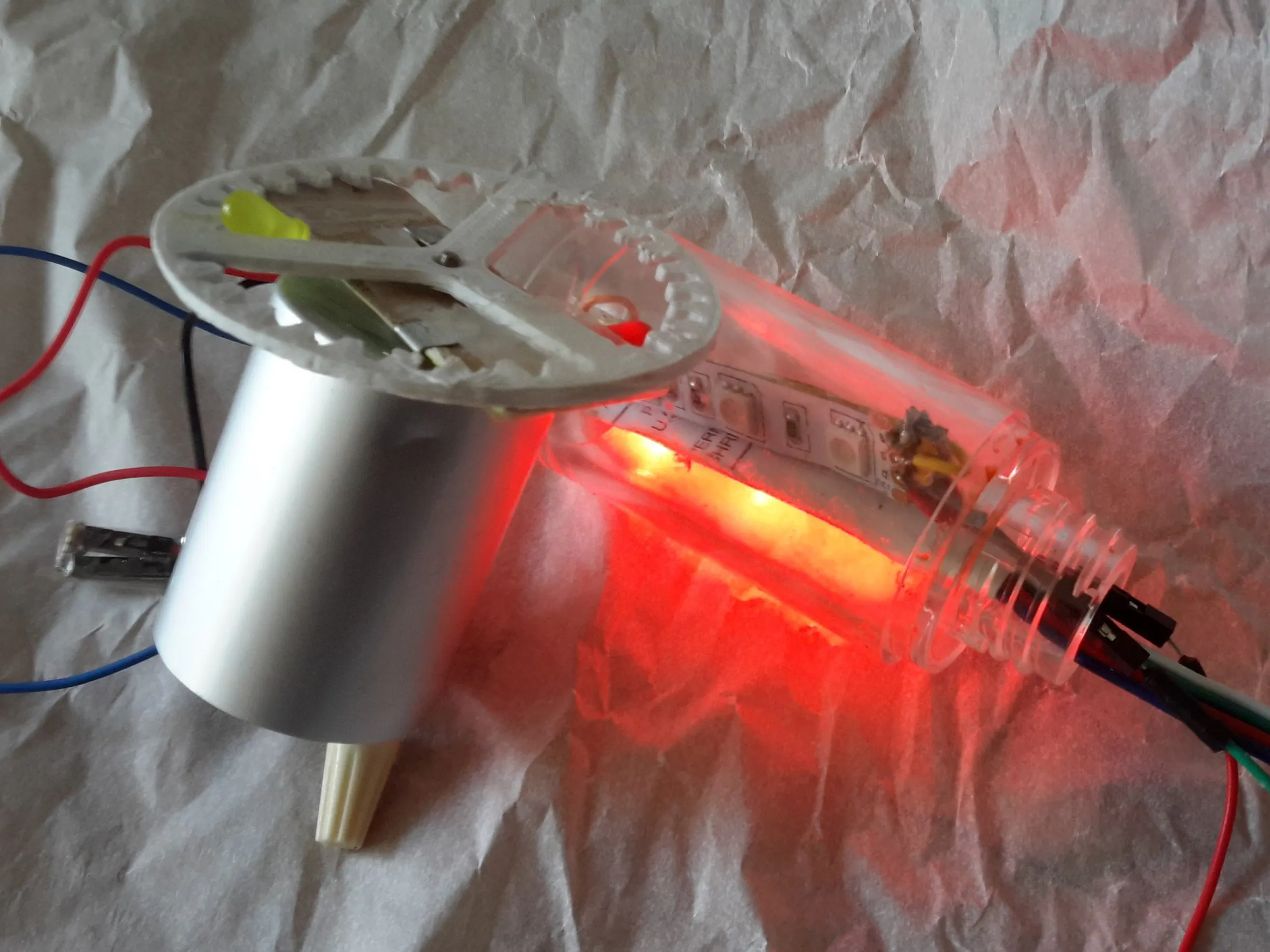## Things used in this project

### Hardware components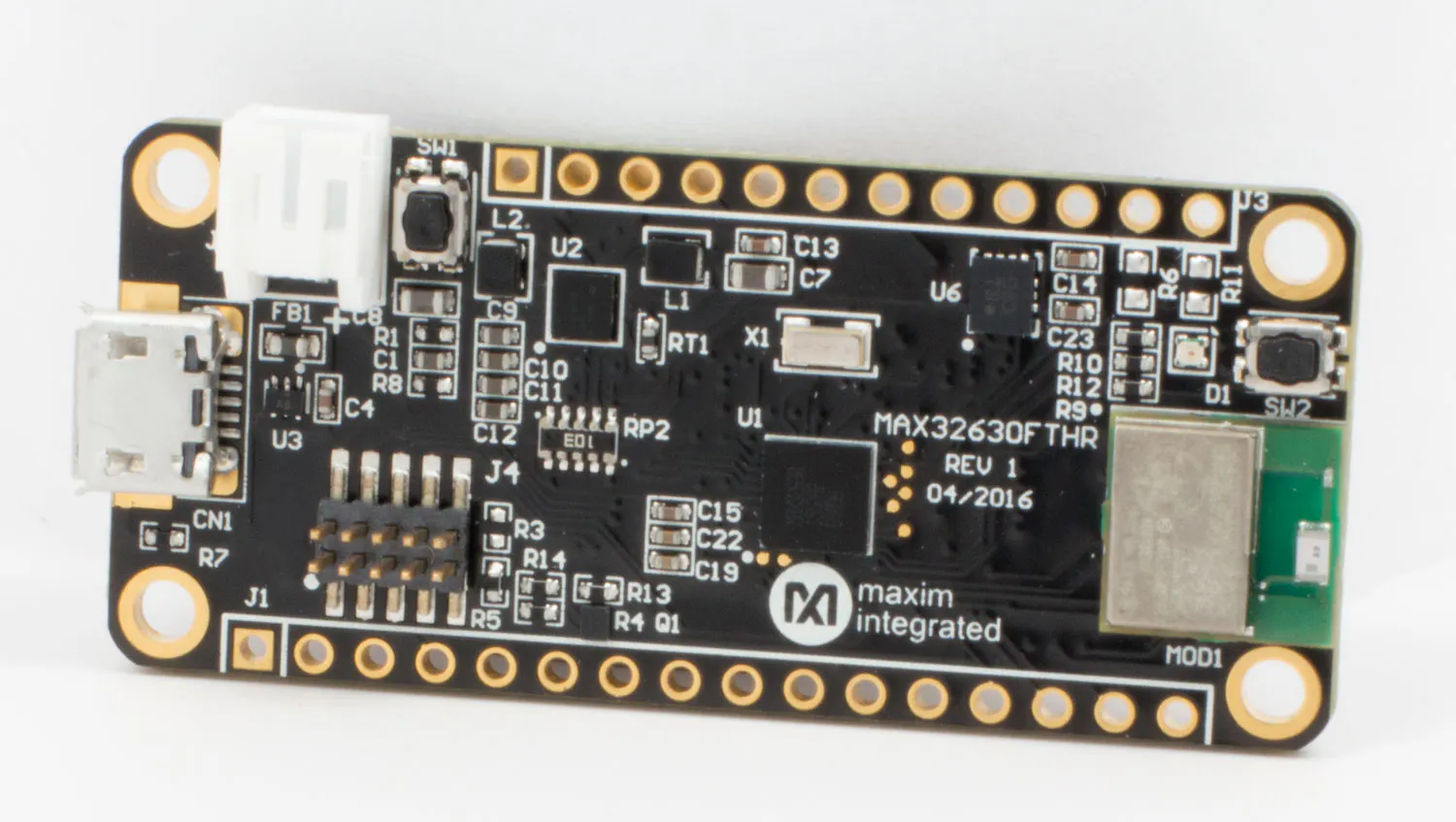Maxim Integrated MAX32630FTHR
×1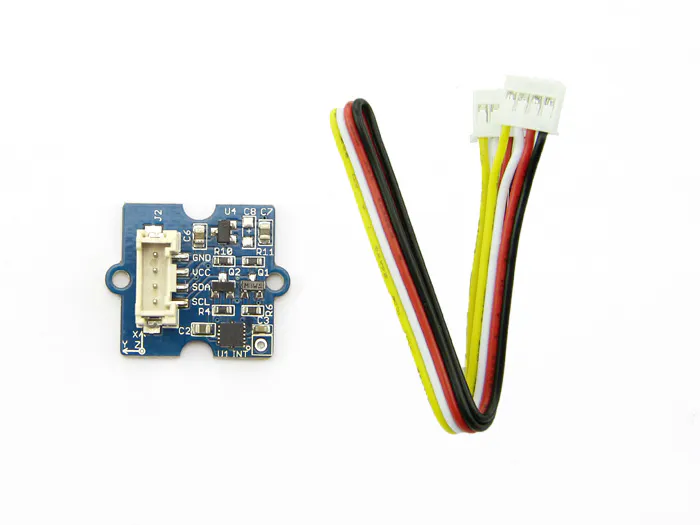Seeed Grove - 3-Axis Digital Accelerometer(±1.5g)
×1
×1
 DC motor (generic)
×1LED (generic)
×2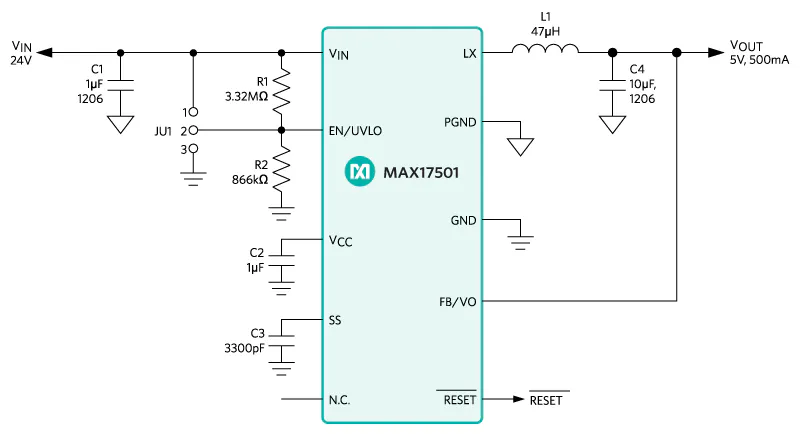Maxim Integrated MAX17501 60V, 500mA, Ultra-Small, High-Efficiency, Synchronous Step-Down DC-DC Converter
×1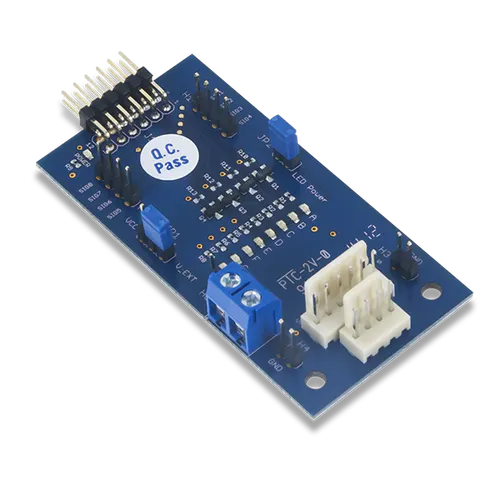Digilent Pmod STEP
×1Resistor 10k ohm
×2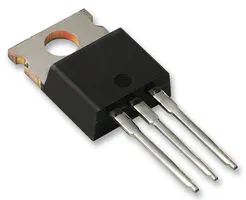Darlington High Power Transistor
×3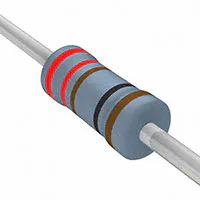Resistor 221 ohm
×1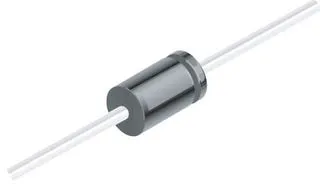1N4007 – High Voltage, High Current Rated Diode
×1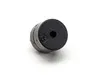Buzzer
×1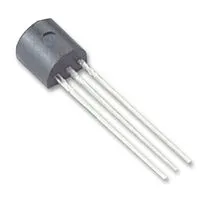General Purpose Transistor NPN
×2

 pliers

## Schematics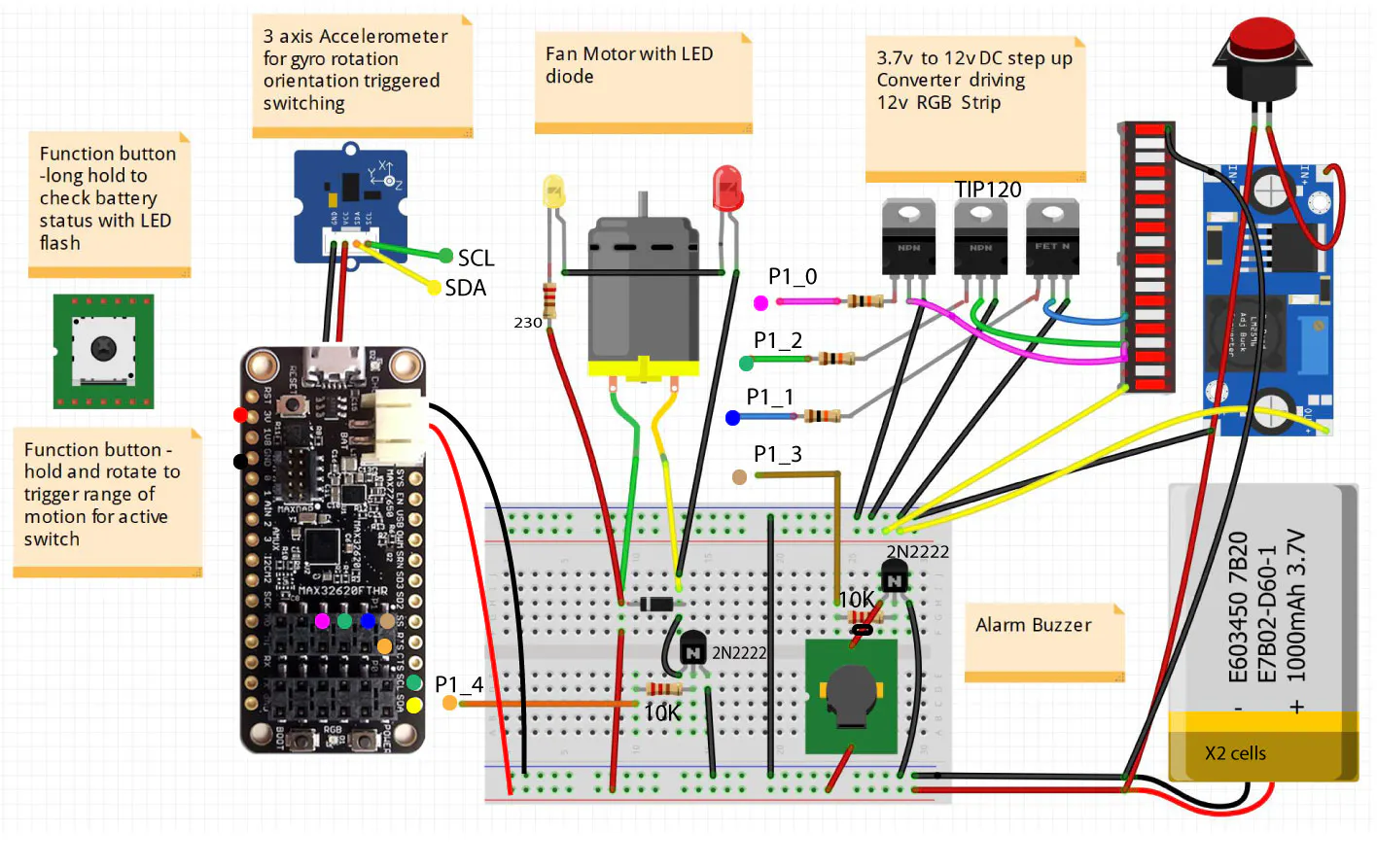## Code

### Light Stick Controller Code

C/C++
See complete project in MBED site
```#include "mbed.h"
#include <float.h>
#include "MAX17055.h"
// I2C Master 2
I2C i2c(I2C2_SDA, I2C2_SCL);
MAX17055 max17055(i2c);

// 3 Axis Accelerometer
#include "MMA7660FC.h"

Serial pc(USBTX, USBRX);

// On board color LED
#include "MAX326XXFTHR_PwmOut.h"

MAX326XXFTHR_PwmOut led[] = {
MAX326XXFTHR_PwmOut(LED2),
MAX326XXFTHR_PwmOut(LED3),
MAX326XXFTHR_PwmOut(LED1)
};

//MAX326XXFTHR_PwmOut motor =  MAX326XXFTHR_PwmOut(P1_0);
DigitalOut motor(P1_4, 0);
DigitalOut buzzer(P1_3,0);

// External RGB LED controll
DigitalOut LED_R(P1_0);
DigitalOut LED_G(P1_2);
DigitalOut LED_B(P1_1);

/*
#include "PololuLedStrip.h"
PololuLedStrip ledStrip(P1_3);
#define LED_COUNT 8
rgb_color colors[LED_COUNT];
*/

DigitalIn inputButton(P2_7); // Input Boot button
DigitalIn button2(P1_5);
DigitalOut power_Hold(P2_2, 1); // Keep power on battery use

int status;
int count = 0, value, max, min;
float f_value, f_max, f_min;

Timer timer;

/*
// Converts a color from the HSV representation to RGB.
rgb_color hsvToRgb(float h, float s, float v)
{
int i = floor(h * 6);
float f = h * 6 - i;
float p = v * (1 - s);
float q = v * (1 - f * s);
float t = v * (1 - (1 - f) * s);
float r = 0, g = 0, b = 0;
switch(i % 6){
case 0: r = v; g = t; b = p; break;
case 1: r = q; g = v; b = p; break;
case 2: r = p; g = v; b = t; break;
case 3: r = p; g = q; b = v; break;
case 4: r = t; g = p; b = v; break;
case 5: r = v; g = p; b = q; break;
}
return (rgb_color){r * 255, g * 255, b * 255};
}
*/

void setColor(int r, int g, int b) {
led.write(1-r);  led.write(1-g);  led.write(1-b);
LED_R = (r > 0.5)?1:0;  // TODO - repalce with PWM
LED_G = (g > 0.5)?1:0;
LED_B = (b > 0.5)?1:0;
}

// Show battery status light using internal LED
// Color indicates estimated capacity based on voltage level
// battery drain value -1 to -100 . pluged in charging 0.4max mw
void showBatteryStatusLight() {
float volt = max17055.avg_v_cell(&f_value);
float current = max17055.current(&f_value);
float pause_time_ms = 400+ current*100;  //TODO- adjust calculated value
int flash_count = 3;

if (current == 0 && volt == 0 ) {
return;
}

//Charging via usb
if (current > 0) {
flash_count = 6;
pause_time_ms = 0;
}

// Flash led
for (int c = flash_count; c > 0 ; c--) {
if (volt >= 4)
setColor(0,0,1);  //blue
else if (volt >= 3.6)
setColor(0,1,0);  //green
else if (volt >= 3.45)
setColor(0.5,0.5,1); //yellow
else
setColor(1,0,0); // red

wait_ms(150);
setColor(0,0,0);
if (pause_time_ms > 0)
wait_ms(pause_time_ms );
}
}

void showBatteryStatus() {
pc.printf("Voltage ");

// Print formatted voltage, current and temperature values
max17055.v_cell(&f_value);
pc.printf("%6.3fV ", f_value);
max17055.avg_v_cell(&f_value);
pc.printf("%6.3fVavg ", f_value);
max17055.max_min_volt(&f_max, &f_min);
pc.printf("%6.3fVmax %6.3fVmin\r\n", f_max, f_min);

max17055.current(&f_value);
pc.printf("%6.3fI ", f_value);
max17055.avg_current(&f_value);
pc.printf("%6.3fIavg ", f_value);
max17055.max_min_curr(&f_max, &f_min);
pc.printf("%6.3fImax %6.3fImin\r\n", f_max, f_min);

max17055.temp(&f_value);
pc.printf("%6.3fC ", f_value);
max17055.avg_ta(&f_value);
pc.printf("%6.3fCavg ", f_value);
max17055.max_min_temp(&f_max, &f_min);
pc.printf("%6.3fCmax %6.3fCmin\r\n", f_max, f_min);
pc.printf("\r\n");
showBatteryStatusLight();
}

#define DEGREE_OFFSET 15
float maxX=FLT_MAX  , minX=FLT_MIN;
float maxY=FLT_MAX, minY=FLT_MIN;
float maxZ=-45, minZ=45;
bool calibrate = false;

bool RotationInRange(float x, float y, float z) {
return !(x > maxX || x < minX ||
y > maxY || y < minY ||
z > maxZ || z < minZ);
}

int main()
{
pc.baud (9600);

// initialize 3 axis accelerometer
Acc.init();                                                     // Initialization
pc.printf("Value reg 0x06: %#x\n", Acc.read_reg(0x06));         // Test the correct value of the register 0x06
pc.printf("Value reg 0x08: %#x\n", Acc.read_reg(0x08));         // Test the correct value of the register 0x08
pc.printf("Value reg 0x07: %#x\n\r", Acc.read_reg(0x07));       // Test the correct value of the register 0x07

// Set sense resistor value
max17055.init(0.05f);

//Show battery gauge status
max17055.status(&status);
pc.printf("MAX17055 status: %04X\r\n", (uint16_t)status);
setColor(1,0,0);

timer.start();

float x=0, y=0, z=0;

while(1) {

// get input button state
//if (inputButton  > 0 || button2  > 0)
//  calibrate = true;

if (time % 100 == 0) {
//pc.printf("Tilt %2.2f  %2.2f  %2.2f\n",x,y,z);

if  (calibrate) {
// Get active acclereometer rotational values and set min max range

if (x > maxX) maxX = x;
if (y > maxY) maxY = y;
if (z > maxZ) maxZ = z;

if (x < minX) minX = x;
if (y < minY) minY = y;
if (x < minZ) minZ = z;
continue;
}

//Accelerometer controlled switching demo
//TODO - replace with input button control

// Sound audio alarm if not in range of active rotation
if ( !RotationInRange(x,y,z))  {
pc.printf("B ");
buzzer = 1;
wait(1);
buzzer = 0;
}

// Control Motor
if (x <= DEGREE_OFFSET && x >= -DEGREE_OFFSET)
setColor(1,0,0);
motor= 1;
wait(1);
} else
motor =0;

// Show Battery Status
if (y <= DEGREE_OFFSET && y >= -DEGREE_OFFSET) {
setColor(0.5,0,0.5);
// showBatteryStatusLight();
}

// Turn on white led lights
if (z <= DEGREE_OFFSET && z >= -DEGREE_OFFSET) {
pc.printf("W ");

setColor(1,1,1);
wait(1);
}

// Change display LED color based on orientation

setColor(  (x+90)/180, (y+90)/180,(z+90)/180 );

/*
// Update LED Strip
for(int i = 0; i < LED_COUNT; i++)
{
uint8_t phase = (time >> 4) - (i << 2);
colors[i] = hsvToRgb(phase / 256.0, 1.0, 1.0);
}
// Send the colors to the LED strip.
ledStrip.write(colors, LED_COUNT);
*/

// Reset timer and show full debug Battery Status info
if (time > 30000) {
showBatteryStatus();
timer.reset();
}
}
}

```

## Credits

### Leon Chu

11 projects • 13 followers
Indie Developer / Artist / Lifelong learner